# AFOQT: Circles & Triangles Chapter Exam

Exam Instructions:

Choose your answers to the questions and click 'Next' to see the next set of questions. You can skip questions if you would like and come back to them later with the yellow "Go To First Skipped Question" button. When you have completed the practice exam, a green submit button will appear. Click it to see your results. Good luck!

### Page 1

#### Question 1 1. Which theorem or postulate can be used to establish congruence with the pictured triangles?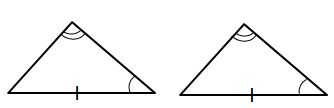#### Question 3 3. If the pictured triangles are congruent, what reason can be given?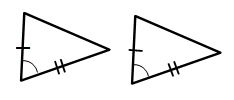### Page 2

#### Question 6 6. Which theorem or postulate can be used to establish congruence with the pictured triangles?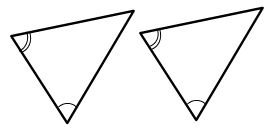#### Question 7 7. If angle a is 80 degrees, what is the measure of angle d?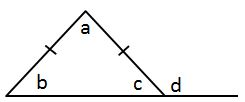#### Question 8 8. What type of triangle is pictured?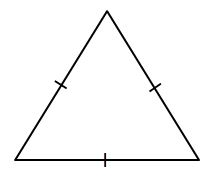#### Question 9 9. Which theorem or postulate can be used to establish congruence with the pictured triangles?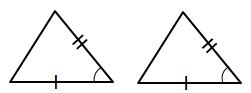### Page 3

#### Question 11 11. In the picture below, if arc AC is 146 degrees and arc BD is 32 degrees, what is the measure of angle AEC?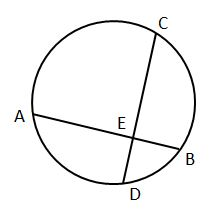#### Question 12 12. What type of triangle is pictured?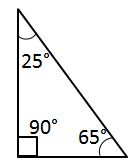#### Question 13 13. In the figure below, Angle 13 = 10y and Angle 11 = 3y + 11. Determine the measure of Angle 16.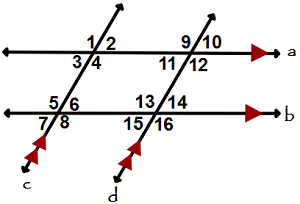### Page 4

#### Question 17 17. Given the triangle ABC, solve for the value of y.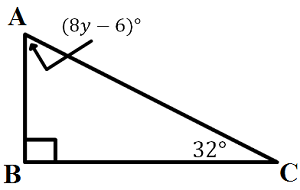#### Question 18 18. If angle ACB is 47 degrees, what is the measure of angle AOB?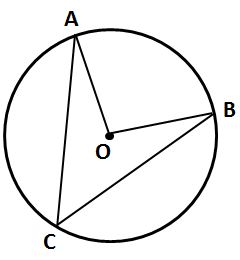#### Question 19 19. What additional information do you need to prove that triangle ABC is congruent to triangle DEF using the HA theorem?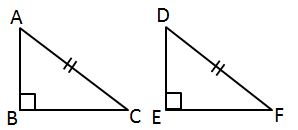#### Question 20 20. If angle a is 30 degrees and angle d is 70 degrees, what is the measure of angle b?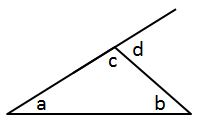### Page 5

#### Question 24 24. In the picture below, if arc CE is 172 degrees and arc BD is 80 degrees, what is the measure of angle CAE?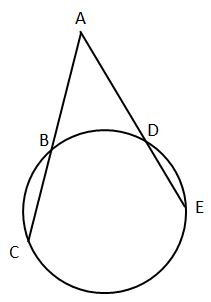#### Question 25 25. In the pictured triangle, what is side B, relative to angle x?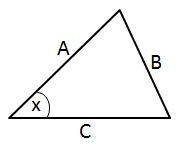### Page 6

#### Question 26 26. What type of triangle is pictured?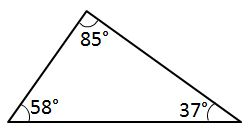#### Question 29 29. What additional information do you need to prove that triangle ACD is congruent to triangle DBA using the HL theorem?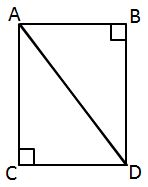#### Question 30 30. What additional information do you need to be given to prove that triangle PQS is congruent to triangle SRP using the HA theorem?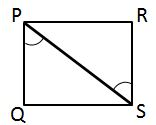#### AFOQT: Circles & Triangles Chapter Exam Instructions

Choose your answers to the questions and click 'Next' to see the next set of questions. You can skip questions if you would like and come back to them later with the yellow "Go To First Skipped Question" button. When you have completed the practice exam, a green submit button will appear. Click it to see your results. Good luck!

Support